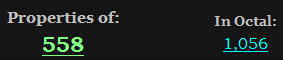CipherJan 29, 2017

Trump signs executive actions on lobbying, ISIS, NSC

http://edition.cnn.com/2017/01/28/politics/donald-trump-executive-actions/index.htmlTrump signs executive actions on lobbying, ISIS, NSC 216 (K/V Exception)
6*6*6 = 216
Trump signs executive actions on lobbying, ISIS, NSC = 558 (Ordinal)
Saturn = 114+6+120+126+108+84 = 558 (Sumerian)156th prime number is 911 a reflection of 119 (God Saturn = 119, All seeing eye = 119, Star of David = 119)
Trump  won the election vote on November Ninth (11/9)
November Ninth = 40+50+700+5+30+2+5+80 + 40+9+40+100+8 = 1109 (Jewish)
November Ninth = 50+60+400+5+40+2+5+90 + 50+9+50+200+8 = 969 (English)
17th tetrahedral number is 969 - the speed of Saturn is 9.69km/s according to our beloved scientism priests
God = 17, EL = 17 (Saturn is known as EL)

This comes on 1/28/2017 - 1+28+2+0+1+7 = 39 - reflection of 93(Saturn) - There's 39 books in the Old Testament that Jews go by, which clearly worships Saturn (EL)
1/28/2017 - 1+2+8+2+0+1+7 = 21 (Saturn = 21 - Reduction)
1/28/2017 - 1+28+20+17 = 66 , Thirty-three = 66(R)/156(Ordinal)The video on this post is 509 seconds long and there will be some 59's coming later in the postThe first line has such nice gematria to open the postTrump's tombstone appeared in Central Park 227 days before he won the election on 314th day in the year, 22/7 = 3.147x7x7 = 343
Twenty-two divided by seven = 314 (Ordinal)
Seven hundred seventy seven = 314 (Ordinal)
Weapons of Mass Destruction = 314 (Ordinal)

Plan on how to defeat ISIS is going to be delivered to Trump 'within 30 days'
Thirty days = 2+8+9+9+2+7 + 4+1+7+10 = 59 (S Exception)
Plan to defeat ISIS = 60+20+1+40 + 100+50 + 4+5+6+5+1+100 + 9+90+9+90 = 590 (Jewish)
Kill = 10+9+20+20 = 59 (Jewish)Michael Flynn = 4+9+3+8+1+5+3 + 6+3+7+5+5 = 59 (Reduced)
Tom Bossert = 2+6+4 + 2+6+1+1+5+9+2 = 38 (Reduced)
Death = 4+5+1+20+8 = 38 (Ordinal)
Killing = 2+9+3+3+9+5+7 = 38 (Reduced)

I think it's going to be very successful = 411 (Ordinal)
Assassin = 1+90+90+1+90+90+9+40 = 411 (Jewish)
They end the post with "That's big stuff"
That's big stuff = 2+8+1+2+1 + 2+9+7 + 1+2+3+6+6 = 50 (Reduced)
Thirty days = 2+8+9+9+2+7 + 4+1+7+1 = 50 (Reduced)
America = 1+13+5+18+9+3+1 = 50 (Ordinal)